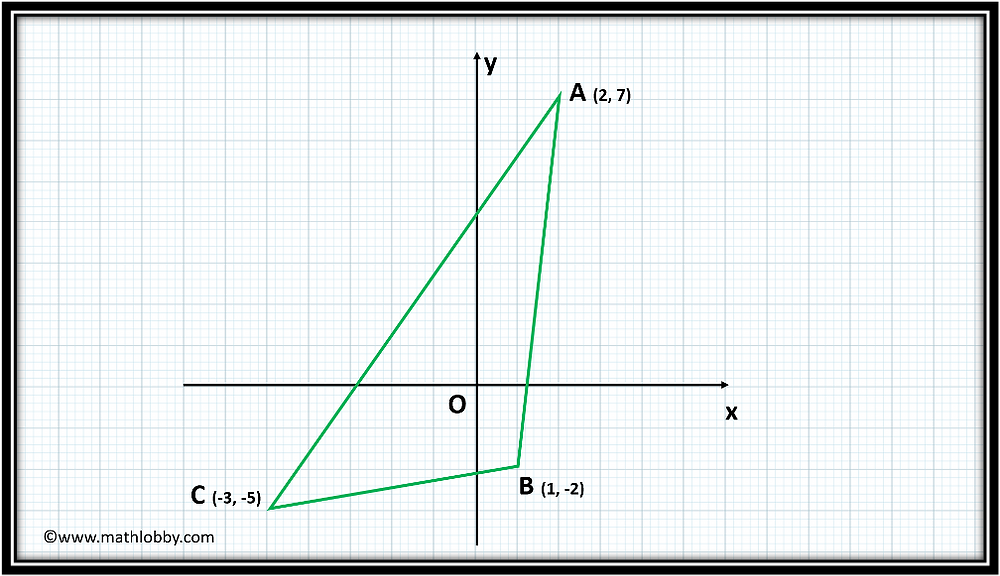Search

# How To Find Area Of Triangles Without Perpendicular Base Or Height

Updated: Jul 1, 2021

## How to find the area of triangles without perpendicular base or heightFrom the diagram shown above, we learnt in past chapters that we can find the area of a triangle using the formula:

### Area of triangle = ½ x base x height

This is applicable for the right-angled triangle ABC, since the length of its base and height can be found in a straightforward manner i.e. parallel to the x and y-axis.

However, for triangle DEF, the length of its base and height are not as clear-cut as for triangle ABC. So, how do we find the area of triangle DEF? In this chapter, we will be learning a new formula to calculate the area of any triangles when we are given the coordinates of all its vertices. Let’s begin!From the diagram above, you can see the triangle ABC such that A (x1, y1), B (x2, y2) and C (x3, y3) are in an anticlockwise direction.

We can see that: Area of triangle ABC = Area of trapezium BMLA + area of trapezium ALNC – area of trapezium BMNC

= [½ (y1 + y2) (x1 – x2)] + [½ (y1 + y3) (x3 – x1)] – [½ (y2 + y3) (x3 – x2)]

= ½ [(x1y1 – x2y1 + x1y2 – x2y2) + (x3y1 – x1y1 + x3y3 – x1y3) – (x3y2 – x2y2 + x3y3 – x2y3)]

= ½ (x1y2 + x2y3 + x3y1 – x2y1 – x3y2 – x1y3)

= ½ [(x1y2 + x2y3 + x3y1) – (x2y1 + x3y2 + x1y3)]

If you find the above formula complex, don't worry! The above formula can be simplified as:Where the products taken in the direction pointed by the black arrows are given positive signs,

i.e. x1y2 + x2y3 + x3y1

and the products taken in the direction pointed by the blue arrows are given negative signs.

i.e. -x2y1 - x3y2 - x1y3 or – (x2y1 + x3y2 + x1y3)

Therefore, if A (x1, y1), B (x2, y2) and C (x3, y3) are in an anticlockwise direction, then the formula above holds true.

Note: This formula is only applicable when the vertices are taken in the anticlockwise direction. If the vertices are taken to be in a clockwise direction, then the resultant value obtained will hence be negative.

Let’s take a look at the application of this formula in the example question shown below:

· Find the area of the triangle with vertices A (2, 7), B (1, -2) and C (-3, -5).

Solution:Area of triangle ABC = ½ {[(2) (-5) + (-3) (-2) + (1) (7)] – [(7) (-3) + (-5) (1) + (-2) (2)]}

= ½ [(-10 + 6 + 7) – (-21 – 5 – 4)]

= ½ [(3) – (-30)]

= 16.5 units^2

And that’s all for today, students! If you have any pending questions, please do go on to our Facebook page, Instagram or contact us directly at Math Lobby! We have certified mathematics tutors to aid you in your journey to becoming a better student!

As always: Work hard, stay motivated and we wish all students a successful and enjoyable journey with Math Lobby!